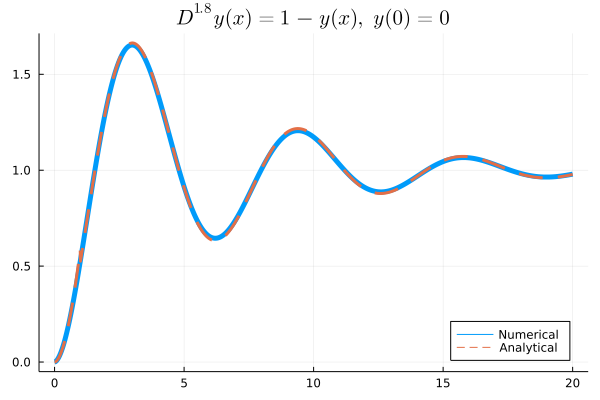# Get Start

We would use a simple example – Relaxation Oscillation Process to show you how to use FractionalDiffEq.jl to solve fractional differential equations.🙂

The mathematical model of the fractional order Relaxation Oscillation can be abstracted as an IVP:

$$$D^{1.8}y(t)+y(t)=1,\ (t>0)$$$$$$y^{(k)}(0)=0$$$

While we can know the analytical solution of this equation is:

$$$u(t)=t^{1.8}E_{1.8,\ 2.8}(-t^{1.8})$$$

Here $E_{\alpha, \beta}(z)$ is the Mittag Leffler function.

We can solve this problem by the following code using FractionalDiffEq.jl:

using FractionalDiffEq, Plots
fun(u, p, t) = 1-u
α=1.8; h=0.01; tspan = (0, 20); u0 = [0, 0]
prob = SingleTermFODEProblem(fun, α, u0, tspan)
sol = solve(prob, h, PECE())
plot(sol)

By plotting the numerical result, we can get the approximation result:To provide users a simple way to solve fractional differential equations, we follow the design pattern of DifferentialEquations.jl

## Step 1: Defining a Problem

First, we need to specify the problem we want to solve. Just by passing the parameters —— describing function, orders, initial condition and time span:

using FractionalDiffEq
fun(u, p, t) = 1-u
α = 1.8; u0 = [0, 0]; tspan = (0, 20); h = 0.01;
prob = SingleTermFODEProblem(fun, α, u0, tspan)

The SingleTermFODEProblem is a class of fractional differential equation, describing equations with $D^{\alpha}u=f(t, u)$ pattern. For other patterns and classes of fractional differential equation, please refer to Problem types

## Step 2: Solving a Problem

After defining a problem, we can solve it by calling the solve function:

sol = solve(prob, h, Alg())

Note that there are different algorithms for differential fractional differential equations, such as FODE, FDDE and DODE, we need to choose a suitable algorithm for specific problem. For all the algorithms, please refer to algorithms documentation.

## Step3 : Analyzing the Solution

Simply call plot to visualize the solution:

using Plots
plot(sol)